# Courses

Course: :
Teacher:

### BASIC   ▾  N : 24

Course nameNotePeriodTime
Table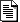Semester I

• Lucia CAPORASO ( caporaso@mat.uniroma3.it - Dipartimento di Matematica e Fisica )

semester I

• Capuano ( laura.capuano@uniroma3.it - Universita degli Studi Roma TRE )Semester ISemester I

• Francesca MEROLA ( merola@mat.uniroma3.it - Dipartimento di Matematica e Fisica )

semester I

• Livia Giacomini ( livia.giacomini@iaps.inaf.it - INAF )
• Cecilia TARANTINO ( tarantino@fis.uniroma3.it - Dipartimento di Matematica e Fisica )

Semester I

• Michela PROCESI ( procesi@mat.uniroma3.it - Dipartimento di Matematica e Fisica )

Semester I

• Gaia CAMISASCA ( gaia.camisasca@uniroma3.it - Dipartimento di Matematica e Fisica )

Semester I

Semester ISemester II -

• Ugo BESSI ( bessi@mat.uniroma3.it - Dipartimento di Matematica e Fisica )

Semester II

• Marco PEDICINI ( marco.pedicini@uniroma3.it - Dipartimento di Matematica e Fisica )

Semester II

• Vincenzo BONIFACI ( vincenzo.bonifaci@uniroma3.it - Dipartimento di Matematica e Fisica )Semester II

• Francesco Cesarone ( francesco.cesarone@uniroma3.it - Universita degli Studi Roma TRE )Semester II

semester II

• Vincenzo BONIFACI ( vincenzo.bonifaci@uniroma3.it - Dipartimento di Matematica e Fisica )

semester II

• Vito Michele ABRUSCI ( michele.abrusci@tlc.uniroma3.it, vitomichele.abrusci@uniroma3.it - Universita degli Studi Roma TRE )Semester II

• Guido GENTILE ( gentile@mat.uniroma3.it - Dipartimento di Matematica e Fisica )

Semester II

Semester II

• Roberto FERRETTI ( ferretti@mat.uniroma3.it - Dipartimento di Matematica e Fisica )

Semester II

RIEMANNIAN GEOMETRY (GE430)

semester II

• Amos Turchet ( amos.turchet@uniroma3.it - Dipartimento di Matematica e Fisica )

Semester II

• Emanuele HAUS ( ehaus@mat.uniroma3.it - Dipartimento di Matematica e Fisica )Semester IISemester II

### INTERMEDIATE   ▾  N : 11

Course nameNotePeriodTime
Table

semester I

• Alexandre STAUFFER ( astauffer@mat.uniroma3.it - Dipartimento di Matematica e Fisica )

Semester I

Semester I

• Luciano TERESI ( luciano.teresi@uniroma3.it - Dipartimento di Matematica e Fisica )

semester I

• ( paolo.delgiudice@roma1.infn.it - Universita La Sapienza )Semester II

Semester II

semester II

• Luciano TERESI ( luciano.teresi@uniroma3.it - Dipartimento di Matematica e Fisica )

semester II

• Roberto MAIELI ( maieli@uniroma3.it - Dipartimento di Matematica e Fisica )

semester II

• Luciano TERESI ( luciano.teresi@uniroma3.it - Dipartimento di Matematica e Fisica )

Semester II

• Severino Angelo Maria BUSSINO ( bussino@fis.uniroma3.it severinoangelomaria.bussino@uniroma3.it sbussino@uniroma3.it - Dipartimento di Matematica e Fisica )

semester II

• Fabio LA FRANCA ( lafranca@fis.uniroma3.it - Dipartimento di Matematica e Fisica )
• Giorgio MATT ( matt@fis.uniroma3.it - Dipartimento di Matematica e Fisica )

### SPECIAL   ▾  N : 11

Course nameNotePeriodTime
Table
PROJECTIVE SPACES AND BIRATIONAL TRANSFORMATIONS (GE520)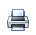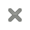#### Projective spaces and birational transformations (GE520)

PROGRAM

1) Linear systems of hypersurfaces in Pn Generalities and basic notions, examples in plane and space geometry.
2) Corrispondences in Pn x Pn Intersection theory, Segre classes, numerical characters of a correspondence. Examples.
2) Cremina transformations Linear system of rational hypersurfaces. Homaloidal linear systems Basic Cremona transformations of Pn.
4) In P2 Quadratic transformations, birational involutions, de Jonquiéres trasformations. Structure of the group. Some finite subgroups.
5) In P3: Linear system of rational surfaces. Transformations defined by quadrics or cubicss. Their inverse transformations.
6) Factorization in P3 Birational maps of P3 and Mori theory. Classification of elementary links with applications. Noether-Fano inequalities. I
teoremi di Max Noether per P2 e di Hilda Hudson per P3. Cenni storici e risultati recenti sul gruppo di Cremona.

- I. Dolgachev ‘Classical Algebraic Geometry’, Cambridge University Press 2012
- I. Dolgachev ‘Lectures on Cremona Transformations, Ann Arbor-Rome’ 2010 -11 http://www.math.lsa.umich.edu/~idolga/cremonalect.pdf
- I. Cheltsov, C. Shramov, ‘Cremona Groups and the Icosahedron’ CRC Press New York 2016
- I. Cheltsov, C. Shramov, Three embeddings of the Klein simple group into the Cremona group of rank three, Transformation Groups
- A. Verra ‘Lectures on Cremona Transformations’, School and Workshop, Torino 2006, pdf.
- Si vedano inoltre le voci ‘Cremona group’ e ‘Cremona transformation’ in Encyclopedia of Mathematics. European Math. Society Press• Alessandro VERRA ( verra@mat.uniroma3.it - Dipartimento di Matematica e Fisica )
KINTIC THEORY OF GASES AND THE KAC MODELApril- May 2022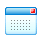• Bonetto Federico ( federicobonetto67@gmail.com - Georgia Institute of Technology, Atlanta )
PROGRAMMING IN PYTHON AND MATLAB (IN400)

semester I• Paola CELIO ( celio@fis.uniroma3.it - Universita degli Studi Roma TRE )
• Sergio Augusto Barcelo Lins ( - Universita La Sapienza )
RATIONAL SIMPLE CONNECTEDNESS IN COMPLEX ALGEBRAIC GEOMETRY#### Rational simple connectedness in complex algebraic geometry

The main goal of this course will be to study a very recent notion in complex algebraic geometry, which generalises rational connectedness: the rational simple connectedness. This notion has, at least conjecturally, striking applications to the existence of rational sections for rationally connected fibrations over surfaces. It is well known that rationally connected fibrations over curves have sections, but the problem becomes much more subtle over higher dimensional base: it is very easy to construct conic bundles over surfaces having no rational sections. Just recently de Jong and Starr introduced the notion of rational simple connectedness for polarised varieties, while looking for sufficient
conditions on the general fibre of a Fano fibration in order to guarantee the existence of a rational section. Rational simple connectedness is the algebraic analogue of simple connectedness in topology: one asks for rational connectedness of certain moduli spaces of rational curves of sufficiently high degree.

In this course I will discuss the following topics:

1. rational curves on Fano varieties;
2. rational simple connectedness of complete intersections;
3. rational simple connectedness of rational homogeneous varieties and Serre's conjecture II;
4. rational simple connectedness and higher Fano varieties.

June-July 2022• Fanelli Andrea ( andrea.fanelli.1@u-bordeaux.fr - Institut de Mathématiques de Bordeaux, France )
STACKS AND MODULI#### STACKS AND MODULI

The course will give an introduction to the theory of algebraic stacks,
focusing on its application to moduli theory.
We start by giving motivation and an overview of the program, which
will follow mostly Jarod Alper's notes Notes on Stacks and moduli.
According to the interests of participants, further topics can be cov-
ered in the last part of the course.
The course will be mostly covered by lectures from participants, ac-
cording to the program and references for each lecture.
1. Program
 Motivation and overview of the program. Guiding examples:
the moduli spaces of curves and the moduli spaces of vector
bundles on a curve.
 Basics on category theory. Sheaves and Grothendieck topolo-
gies.
 Groupoids and pre-stacks.
 Stacks
 Algebraic spaces and algebraic stacks
 Geometric properties of algebraic stacks
 Characterization of Deligne-Mumford stacks
 Geometry of Deligne-Mumford stacks
 Existence of coarse and good moduli spaces
 The moduli stack of curves
 Stacks on di erent geometric contexts (topological stacks, cone
stacks, etc)
2. References
(1) Notes on stacks and moduli, Jarod Alper
(2) Champs algebriques, Laumon and Moret-Bailly
(3) Notes on Grothendieck topologies, bered categories and descent
theory, Vistoli
(4) Algebraic spaces and stacks, Olsson
(5) The Stacks Project
(6) Lecture notes on moduli theory, Halpern-Leistner
(7) Existence of moduli spaces for algebraic stacks, Alper, Halpern-
Leistner, Heinloth
(8) The irreducibility of the space of curves of given genus, Deligne
and Mumford
(9) Moduli of Curves, Harris and Morrison
(10) Projectivity of complete moduli, Kollar
1

March - May , 2022HANDS ON CONTINUUM MECHANICS (MINI COURSE)#### Hands on Continuum Mechanics (mini course)

Goal: understand the fundamentals of continuum mechanics through worked examples. Participants will tackle some typical problems of continuum mechanics, and will learn to implement a problem using the weak formulation into the COMSOL software and to discuss the solution.

Synopsis of lectures
1) Browse a model of nonlinear solid mechanics, from the implementation to the solution.
A first glance at the fundamentals of continuum mechanics: Kinematics, Constitutive, Balance laws.
Tell the difference between tensors: strain tensor versus stress tensor
Differential form (strong) versus Integral form (weak).

2) Material Versus Spatial description.
A continuum body as a differentiable manifold.
Pull back & push forward of scalar, vector and tensor fields.
Geometric elements; change of densities.

3) Solid mechanics versus Fluid mechanics
Kinematical constraints; isochoric motion.
Reference stress (Piola) & Actual stress (Cauchy).
Polar decomposition theorem; eigenspace of the stress tensor and of the strain tensor

4) Non linear solid mechanics
Worked example: large deformations of a hyperelastic solid under and distortions. Target metric.

5) Material response
Worked example: from elastic energy  to the constitutive law for the stress.
Transversely isotropic materials. Fiber reinforced materials.
Worked example: fiber reinforced hyperelastic solid under traction.

6) Fluid dynamics
Tackling Navier Stokes equations.
Worked examples: fluid in a channel; fluid around an obstacle.

7) Fluid-Structure interactions - theory
Worked examples: understand the moving mesh technique; how to write the problem of a beam immersed in a fluid.

8) Fluid-Structure interactions - practice
Worked example: implement and solve the problem of an oscillating beam immersed in a fluid.

March 2022• Luciano TERESI ( luciano.teresi@uniroma3.it - Dipartimento di Matematica e Fisica )
INTRODUCTION TO EXPANSIONS IN NON-INTEGER BASES#### Introduction to expansions in non-integer bases

1. This theory grew out of an example in a historical paper of A. R ?enyi in 1956,
illustrating the use of ergodic theory in investigating the frequency of digits, a problem going back to a famous theorem of Borel. Subsequent research revealed many hitherto hidden connections of his example to number theory, topology, fractals, symbolic dynamics and to probability. The theory got a new impetus with the discovery in 1990 of P. Erdo ?s and his collaborators of strange and unexpected properties of unique
expansions. This led to hundreds of papers in the past thirty years. We propose an introduction to this subject, which is still rich in open problems.

The plan of our course is the following:
1. (i)  Definition of expansions, examples illustrating the differences between integer and non-integer bases.
2. (ii)  Lexicographic characterizations.
3. (iii)  The number of expansions.
4. (iv)  Univoque bases.
5. (v)  Topological description of the set of numbers having a unique expansions.
6. (vi)  Hausdorff dimension of the preceding set.
7. (vii)  Multiple expansions.
---------------------------
References:
• A. R´enyi, Representations for real numbers and their ergodic properties, Acta
Math. Hungar. 8 (1957) 477–493.
• P. Erd?os, I. Jo´o, P V. Komornik, Characterization of the unique expansions 1 =
1i
=1 q−ni and related problems, Bull. Soc. Math. France 118 (3) (1990) 377–
390.
• V. Komornik, P. Loreti, Unique developments in non-integer bases, Amer. Math.
Monthly 105 (7) (1998) 636–639.
• M. de Vries, V. Komornik, Unique expansions of real numbers, Adv. Math. 221
(2) (2009) 390–427.
• V. Komornik, A. C. Lai, M. Pedicini, Generalized golden ratios of ternary alphabets,
J. Eur. Math. Soc. (JEMS), 13 (4) (2011) 1113–1146.
• V. Komornik, D. Kong, W. Li, Hausdor↵ dimension of univoque sets and devil’s
staircase, Adv. Math. 305 (2017) 165–196.

May 2022APPLICATIONS OF FOURIER ANALYSIS IN THE THEORY AND CONTROL OF PDES#### Applications of Fourier analysis in the theory and control of PDEs

Fourier analysis is historically strongly linked to the resolution of PDEs with the fundamental example of the heat equation that was initially introduced by Fourier himself. In this course, we will first give an introduction of the different concepts entering in the Fourier analysis.
Then, we will give a general framework based on Fourier analysis, to solve classical PDEs. We will consider as fundamental examples, the heat and the wave equations, and answer the question of existence and unicity of the solution in appropriate function spaces. The we will deal with more advanced topics. The first one is linked to the finding of
linearized solutions of the Vlasov equation that enter in the kinetic description of the time evolution of a plasma (a typical example is the so called Landau damping that was studied by Cédric Villani in the more difficult case of the non-linear equation). Another topic, is the use of Fourier series to solve some controllability problems. There, the main tool is to obtain generalizations of the Parseval equality that are called Ingham inequalities, which have their own interest.

October 2021• Michel Mehrenberger ( - (Université Aix-Marseille )
AN INTRODUCTION TO CHEEGER'S ENERGY AND ANALYSIS IN METRIC MEASURE SPACES#### An introduction to Cheeger\'s energy and Analysis in metric measure spaces

Many interesting objects, like the Dirichlet

$\frac{1}{2}{\int }_{{\mathbf{R}}^{n}}|\mathrm{\nabla }f{|}^{2}\mathrm{d}x$

energy or the heat equation
${\mathrm{\partial }}_{t}f=\mathrm{\Delta }f$
are defined using the Laplacian or the gradient: at first look, it seems impossible to define them on other spaces than ${\mathbf{R}}^{n}$ ${f R}^n$ .

However, recently the Dirichlet energy has been generalized to Cheeger's energy, which requires only functions defined on a metric space and a Borel measure to calculate the integrals. In this course, we try to give an introduction to Cheeger's energy: we shall give two different definition of Cheeger's energy and prove that they are equivalent. For the proof, we need to introduce the basic definitions and theorems of optimal transport, Wasserstein distance and gradient flows in metric spaces. If there is time, we are going to show a version of the theorem of Otto,  Kinderlehrer and Jordan, which says that the heat equation is the gradient flow of the entropy functional in the metric space of measures with the 2-Wasserstein distance.

Starting January 2022• Ugo BESSI ( bessi@mat.uniroma3.it - Dipartimento di Matematica e Fisica )
GEOMETRY OF PROJECTIVE ALGEBRAIC VARIETIES EXAMPLES, PROBLEMS, EXERCISES#### Geometry of projective algebraic varieties Examples, problems, exercises

Abstract:
The course is an introduction to some beautiful and connected examples, or constructions,
which are relevant in classical, and modern, projective algebraic geometry. The focus will
be on their classical origins and on their importance in current algebraic geometry.
A list of topics and examples to be chosen will be discussed with the students and fixed
according to their interest. This possibly includes: Cremona transformations in the space,
line geometry and Grassmannians, projective models of surfaces in P^3, hypersurfaces
of low degree and their unirationality, rationality problems, cubic hypersurfaces, classical
projective examples and hyperkahler varieties..

Starting March 22, 2022• Alessandro VERRA ( verra@mat.uniroma3.it - Dipartimento di Matematica e Fisica )
TOWARDS INFORMATION THEORY, NEURAL NETWORKS, AND BEYOND (READING COURSE)#### Towards Information theory, neural networks, and beyond (Reading course)

Topics:

An introduction to Neural Networks
The single neuron as Classifier
Capacity of a Single Neuron
Learning as Inference
Hopfield Networks
Boltzman Machines
Supervised Learning in Multilayer Networks Deconvolution

Inference from encrypted datasets
crypto keys autonomous learning

Starting November 12, 2021• Marco PEDICINI ( marco.pedicini@uniroma3.it - Dipartimento di Matematica e Fisica )

### IN OTHERS UNIVERSITIES   ▾

Questa pagina è disponibile in: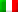Italiano